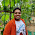## November 01, 2018

Question:
Assume that there are two algorithms A and B for a given problem P. The time complexity functions of algorithms A and B are 5n and log2n    respectively. Which algorithm should be selected assuming that all other conditions remain the same for both the algorithms?

Solution:
Assuming that all conditions remain the same for both algorithms, the best algorithm takes less time when the input is changed to a larger value. Results obtained employing different values of n are shown below:

 Input size (n) Algorithm A T(n) = 5n Algorithm B T(n) = log2n 1 5 0 5 25 2.32 10 50 3.32 100 500 6.64

It can be observed that algorithm B performs better as n is increased; time complexity increases linearly and gives lesser values for larger values of n. First algorithm instead grows exponentially, and 500 is a very large number compared to 6.64. Therefore, algorithm B is better than algorithm A.

Question:

Let us assume that for a telephone directory problem P, three algorithms exist, A, B and C. Time complexities of A, B and C are 3n, 5n and log n respectively. Assume that the input instance n is 10^3. Assume that the machine executes 10^9 instructions per second. How much time will algorithms A, B and C take? Which algorithm will be the best?

Solution:

Here, n = 10^3, and the computer can execute 10^9 instructions per second.

Therefore, algorithm A (time complexity 3n) would take (3 ×10^3) /10^9 = 3/10^6 = 0.000003 seconds.
Algorithm B (time complexity 5n) would take (5 × 10^3)/10^9 = 5/10^6 = 0.000005 seconds.
Algorithm C (time complexity log n) would take (log 10^3) /10^9 = 3/10^6 = 3e-9 seconds.

It can be observed that algorithm C would take very less time compared to algorithm A & B. Hence, it is natural that algorithm C is the preferred one.

Question:

Let N be the number of guests attend a party. If each guest shakes his hand with everyone else only once, how many handshakes will take place? Write a recursive definition and algorithm.

Solution:

The first person may shake hands with n-1 other people. The next person with n-2 other people.
This chain would go as (n-1) + (n-1) + (n-1) + + 2 + 1 = n!

The recursive formula is T(n)=T(n-1)+(n-1) and its Closed formula solution is n*(n-1)/2

Algorithm (n)
Input: n
Output: number of handshakes
Begin number = n*(n-1)/2
return (number)
End

Question:
A Knapsack can carry weights not exceeding 100. The weights and values of of five objects are as follows
Solve the Knapsack problem and find the maximum profit that can be obtained.

Solution:

To solve this problem, you need to understand 3 things:
1.  The maximum weight, a bag can hold.
2.  Select the item which satisfies the first condition and hold the maximum value.
3.  You can not select a same item twice

Focus on below table:

* As per the table, the maximum profit which can be obtained is 143.

Tips: Consider Values as in \$ for each items. You can not select the same item twice. The maximum weight should not exceed 100.

Question:
Consider the following characters { A, B, C, D, * } with the following probability
 Character A B C D * Probability 0.35 0.1 0.2 0.2 0.15

a) Encode the text A B A C A D  using the generated codes
b) Decode text whose coding  is 100 110 111 101 1100

Solution:

C = 00 A = 11 B = 100 D = 01 * = 101
The encoded text A B A C A D Would be {1110011011101}
Decode text 100 110 111 101 1100 Would be B A DA*EAC

Question:
Apply Dijkstra’s algorithm for the following digraph and find the path between nodes A and D.

Solution:

Question:
Solve the following problem using Ford–Fulkerson algorithm.
Source  A , sink  D

Solution:

Construct one augment path ABD. Minimum { 1, 6 } = 1
Next augment path is ACD . Minimum { 3, 8 } = 3
The next augment path is ABCD. Minimum { 5, 5, 5 }=5
Maximum Flow =9
Incidentally the maximum flow is same on the original graph.

Question:
Find the optimal cost and show the final BST tree for four symbols a1, a2, a3, and a4 with the following problem  P1=2/7 \P2 = 1/7  P3  = 1/7 P4 =3/7

Solution:

1.For Encoding and Decoding Question:
2.3.